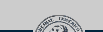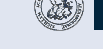# Introduction to Pattern Recognition [IntroPR]

Summary
The goal of this lecture is to familiarize the students with the overall pipeline of a Pattern Recognition System. The various steps involved from data capture to pattern classification are presented. The lectures start with a short introduction, where the nomenclature is defined. Analog to digital conversion is briefly discussed with a focus on how it impacts further signal analysis. Commonly used preprocessing methods are then described. A key component of Pattern Recognition is feature extraction. Thus, several techniques for feature computation will be presented including Walsh Transform, Haar Transform, Linear Predictive Coding, Wavelets, Moments, Principal Component Analysis and Linear Discriminant Analysis. The lectures conclude with a basic introduction to classification. The principles of statistical, distribution-free and nonparametric classification approaches will be presented. Within this context we will cover Bayesian and Gaussian classifiers, as well as artifical neural networks. The accompanying exercises will provide further details on the methods and procedures presented in this lecture with particular emphasis on their application.

Dates & Rooms:
Monday, 10:15 - 11:45; Room: 0.68
Tuesday, 14:00 - 15:00; Room: 01.019

# General Information

A tentative syllabus can be foundhere.

Important Information about the Exam.

A. Because WS 2010 is a transition year for IntroPR and PR, both undergraduates and Master's students are allowed to take the exam. This is only valid for W2010!!

B. Due to a multitude of problems with Mein Campus, IF (and only IF) you have been unable to register for your exam, please register with us (i.e. Elli Angelopoulou or the Secretaries of Informatik 5). You can send us an email or drop by our office. You need to provide the following information:

1. Course you would like to be examined on and for how many credits

2. Full name

3. Studiengang

4. Martikelnummer

5. Birthdate

C. Exam Dates:

Tuesday 22.02.2010

Friday 15.04.2010

You can sign up for a specific date either on Monday 20.12.2010 at the end of the lecture, or on Tuesday 21.12.2010 at the end of the lecture, or anytime after Tuesday's lecture by contacting the secretaries of Informatik 5 via phone, email or in person.

# SlidesIntroduction: course outlineKey PR concepts: the pipeline of a PR system, terminology, postulates of PRSampling: introduction to A/D conversion, Nyquist sampling theoremQuantization: Signal-to-Noise ratio, quantization characteristic funtion, vector quantization, k-means algorithmEqualization and Thresholding: histogram equalization, thresholding, binarization, maximum likelihood estimation, various thresholding algorithms (intersection of Gaussians, Otsu's algorithm, unimodal algorithm, entropy-based)Filtering: Linear Shift Invariant transformations, convolution, mean filter, Gaussian filter, gradient-based edge detector, Laplacian of GaussianNon-linear Filtering: homomorphic filters, cepstrum, morphological operators, rank operatorsNormalization: size, location and pose normalization, geometric momentsIntro to Feature Extraction: curse of dimensionality, heuristic versus analytic feature extraction methods, projection on orthogonal bases, Fourier transform as a featureFeatures based on Projection to Orthogonal Basis: spectrogram, Walsh-Hadamard transform, Haar transformLPC, Moments as Features: Linear Predictive Coding, geometric moments, Hu's set of invariant momentsMultiresolution Analysis: wavelets, continuous wavelet transform, discrete wavelet transform, wavelet seriesPCA, LDA: intro to analytic feature extraction, principal component analysis, eigenfaces, linear discriminant analysis, fisherfacesOFT: optimal feature transform, Mahalanobis distanceOptimization Algorithms: gradient descent, coordinate descentFeature Selection: objective functions for feature selection including conditional entropy and KL-divergence, search strategies, including branch-and-boundIntro to Classification: decision function, statistical classifiers, Bayes classifierGaussian Classifier: Gaussian classifier, linear vs. quadratic decision boundariesPolynomial Classifiers: polynomial classifiers, discriminant functionsNon-Parametric Classifiers: K-nearest-neighbor density estimation, Parzen windowsArtificial Neural Networks: introduction to artificial neural networks (ANN), ANN and classification, radial basis functions (RBF), RBF networksMultilayer Perceptron: feed-forward networks, perceptron, back-propagationRecap: summary of the various topics covered in Introduction to Pattern Recognition

# Videos

All lecture videos should be available via studon. Note that lecture 10 is "intentionally missing" (there was no lecture on Nov. 28th).

\o/